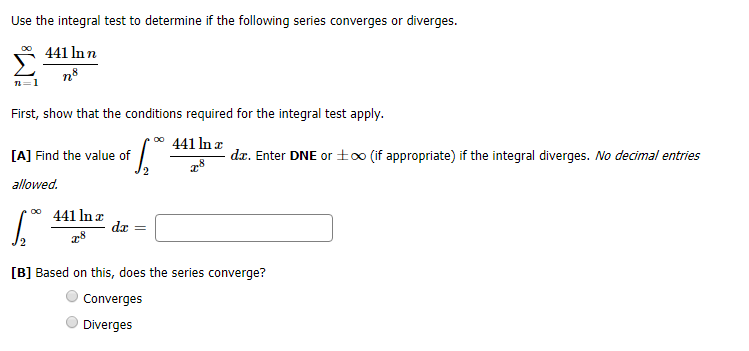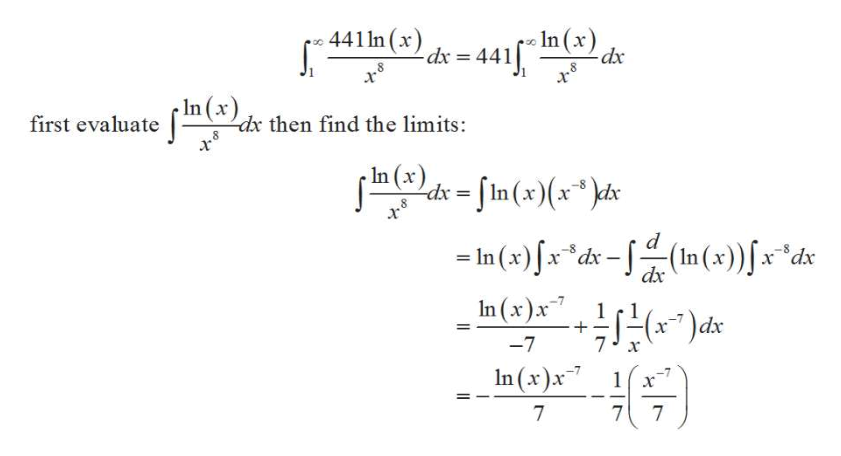# Use the integral test to determine if the following series converges or diverges.441 ln n8ne1First, show that the conditions required for the integral test applyoo441 ln zdz. Enter DNE or±o0 (if appropriate) if the integral diverges. No decimal entries[A] Find the value ofallowed441 In xda[B] Based on this, does the series converge?ConvergesDiverges

Question
1 viewshelp_outlineImage TranscriptioncloseUse the integral test to determine if the following series converges or diverges. 441 ln n 8 ne 1 First, show that the conditions required for the integral test apply oo 441 ln z dz. Enter DNE or±o0 (if appropriate) if the integral diverges. No decimal entries [A] Find the value of allowed 441 In x da [B] Based on this, does the series converge? Converges Diverges fullscreen
check_circle

Step 1

From the given information it is required to determine if the series converges or diverges by using the integral test.

f be a function continuous, positive decreasing on [1,∞) such that an = f(n) then,

Step 2

In the given question the given series and corresponding f(x) is:

Step 3

Now, evaluate the integral to test f...help_outlineImage Transcriptionclose441n (x Lds = 441 (x) dx 8 In dx then find the limits: f(x) first evaluate In (x) d-In(x)(x*) - In (x)x d-(In(x))Jx*a dx In (x)x dx 7 -7 In (x)x 1x 7 7 7 fullscreen

### Want to see the full answer?

See Solution

#### Want to see this answer and more?

Solutions are written by subject experts who are available 24/7. Questions are typically answered within 1 hour.*

See Solution
*Response times may vary by subject and question.
Tagged in

### Other